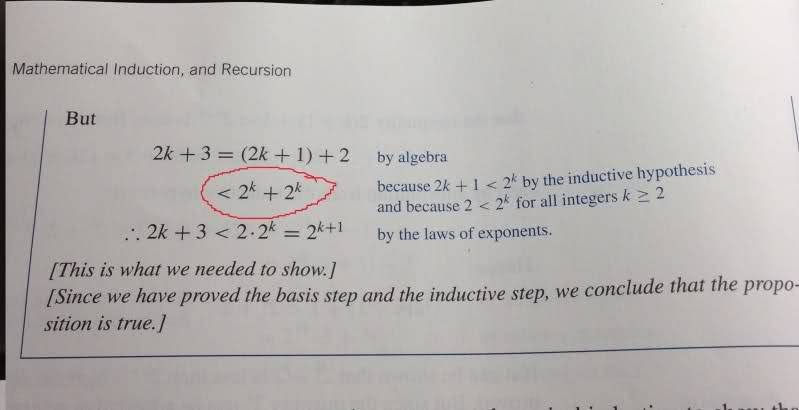# Proof by induction

I am confused by what the book is saying, can someone explain how they got the thing I circled in red?your book explains it on the side, it is using the inductive hypothesis and a fact about integers to say that (2^k+1)+2 < 2^k+2^k=2(2^k)=2^(k+1)

SammyS
Staff Emeritus
Homework Helper
Gold Member
I am confused by what the book is saying, can someone explain how they got the thing I circled in red?
What don't you understand? The thing circled in red is explained just to the right of that.

You're assuming that $2k+1<2^k$ .

It's also true that: $\text{ for }\ k ≥ 2\,,\ \ 2 < 2^k$

Therefore, $2k+1+2<2^k+2<2^k+2^k\,.$

Ray Vickson
Homework Helper
Dearly Missed
I am confused by what the book is saying, can someone explain how they got the thing I circled in red?Their comments in blue tell you exactly how they got it.

RGV

How did they decide 2 < 2k? Where did the 2 come from

they expanded 2(k+1), which is the thing you're trying to prove the relationship about, into (2k+1)+2 on the LHS, and then on the RHS they're just trying to make relatable to 2k+1 so they used the relationship 2 < 2k to facilitate it.

Oh I think I see it now. So when they use 2 < 2k its kinda like stating 2 = 2k so (2k+1) + 2 < 2k + 2k